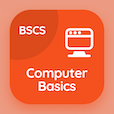Cambridge Online Courses (GCE)

A Level Physics Quizzes

A Level Physics Quiz PDF - Complete

# Orbiting under Gravity Quiz MCQ Online p. 51

Practice Orbiting under Gravity quiz questions and answers PDF, orbiting under gravity trivia questions 51 to learn online A Level Physics course for online classes. Gravitational Field MCQ questions, orbiting under gravity Multiple Choice Questions (MCQ) for online college degrees. "Orbiting under Gravity Quiz" PDF eBook: forces in nucleus, physics experiments, mass and inertia, electromagnetic radiation, orbiting under gravity test prep for accredited online colleges.

"Mass of satellite orbiting Earth is" MCQ PDF: irrelevant, considered, should be infinite, and should be zero for ACT prep classes. Solve gravitational field questions and answers to improve problem solving skills for colleges that offer online courses.

## Trivia Quiz on Orbiting under Gravity MCQs

MCQ: Mass of satellite orbiting Earth is

considered
irrelevant
should be infinite
should be zero

MCQ: Speed of electromagnetic radiation is independent of

wavelength
amplitude
time period
frequency

MCQ: If there is no net force acting on body, then its acceleration is

zero
constant
increasing
decreasing

MCQ: Least count of screw gauge is

0.01 cm
0.5 cm
0.1 cm
0.01 mm

MCQ: Elements undergo radioactive decay when proton number becomes greater than

50
40
83
73

### More Quizzes from A Level Physics Course